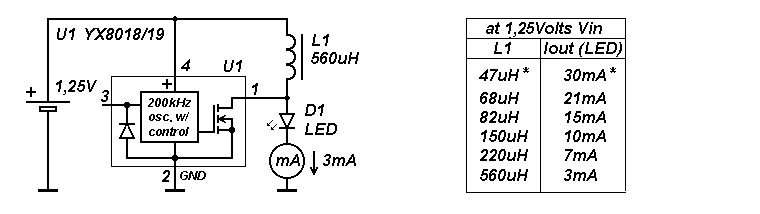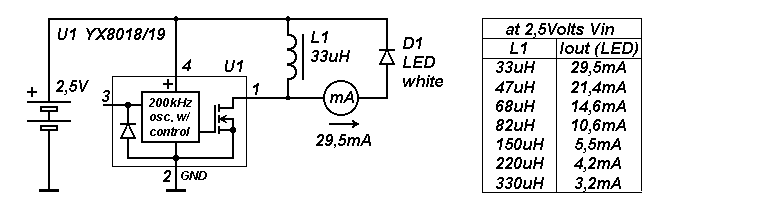17.44.2 Addition, or a simple explanation of why this works.

***We are talking about a simplest L-meter (1-200uH, one range) which was described on this page.

The basic idea is well highlighted in a datasheet for YX8018/8019, which is unexpectedly written in Japanese.
Following the manual, the current through the LED will depend on the coil's inductance and the supply voltage. Assuming that with constant voltage the frequency of the internal generator is constant (which is true), so it should be.
Two different circuits make it possible to obtain a different current through the LED.
In the first, the voltage on the inductor (during the discharge time) is added to the voltage on the battery and increases the output current.At the second, only the energy stored in the inductance is pumped into the LED.***For reference: at 1,25V, this occurs at a frequency of 240 kHz, at 2,5V-200 kHz (fixed pulse width / duty cycle).

The inverse dependence of the output current on the magnitude of the inductance seems wrong, but this is only at first glance. At a fixed voltage around L1 (when the transistor is open), a larger current flows through the smallest inductance and it manages to store more energy in the core. At a constant frequency, this transfers more power (or
current) into the LED.

The output current will depend on the voltage drop across the white LED (~3V at 30mA). For a green LED (voltage drop ~2V/30mA), the output current will be greater. Even more, you can raise the output current by replacing the LED with a Schottky diode (1N5817/19). This will raise the output current (considering the total  voltage drop ~0,3V) up to ~0,3A, for the last circuit.

* Additional current adjustment can be done by changing the supply voltage.
** Calculations are made without taking into count the efficiency of the circuit.

Return to
L-meter  page.
------------------------------------------------------------------------------------------------------------------------------------------

17.04.2017  SKootS

_

Make a free website with Yola